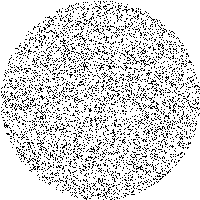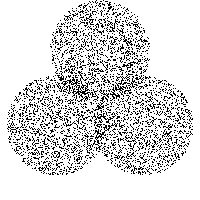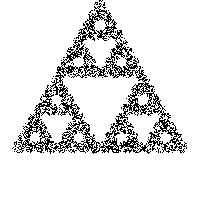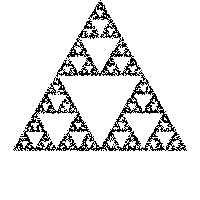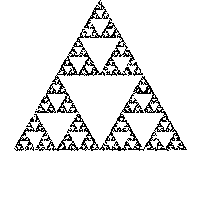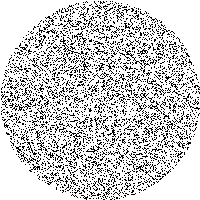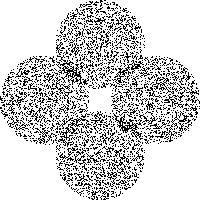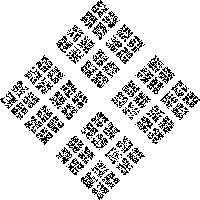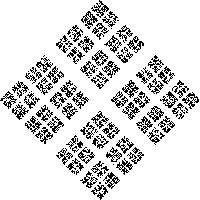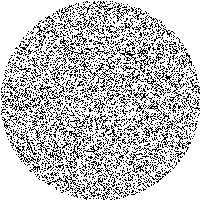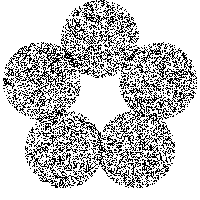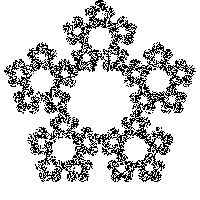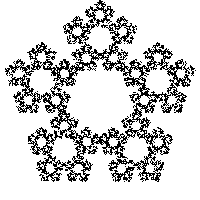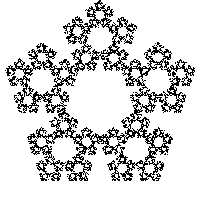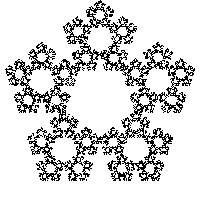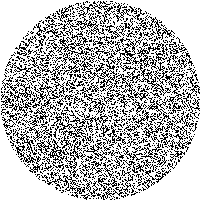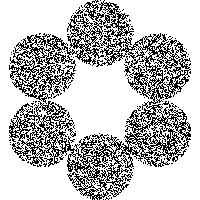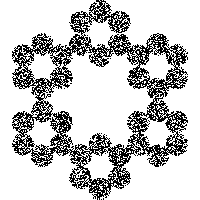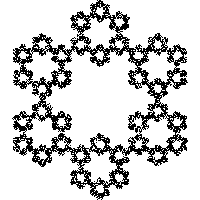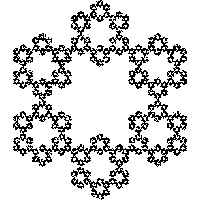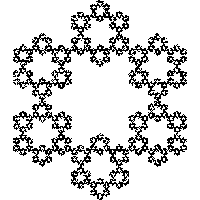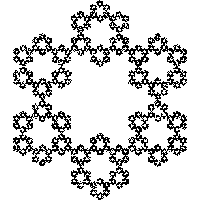# Sierpinski triangle and other shapes

### Rendered in Python, explained in English

##### By Dany Shaanan
Sierpinski triangle is a fractal that can be generated in various ways. One of these ways is by pulling a set of points towards the vertices of a triangle in the following way:

A set of random points is generated, as seen in the first row of images.

Those points are devided randomly into three sets coresponding to the three vertices of the triangle, and each point moves half of its way to that vertex. This can be seen in the first image of the second row.

This process is repeated, each time redeviding the points into sets. The left-most column show what happens when this is done, as it is usually, on a triangle. The other columns shows the same process on a square, a pentagon, and a hexagon.

See the code here